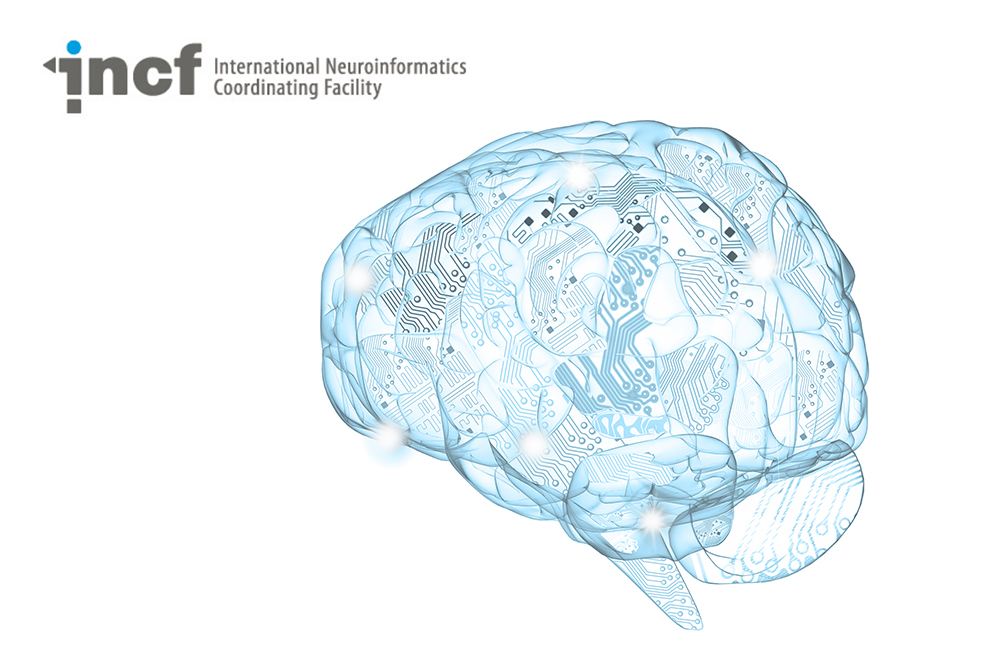# Basic mathematics for computational neuroscience

Category
Level
Beginner

A series of short explanations of the basic equations underlying computational neuroscience.

Membrane potential equation
Separation of variables
Injecting current into a passive membrane
Response to injected current
Voltage-dependent ion channels into the passive membrane
Hodgkin & Huxley's voltage dependent ion channel models
Classical Hodgkin & Huxley squid axon model
Multi-compartment conductance-based models
Lessons of this Course
1
Duration:
08:16

Introduction to the membrane potential equation

2
Duration:
9:22

Explanation of the equivalent circuit model for a patch of passive neural membrane.

3
Duration:
4:04

Solving the passive membrane equation

4
Duration:
5:54

Injecting current into a passive membrane

5
Duration:
7:34

Response to injected current

6
Duration:
9:55

Explains the logic behind dealing with more complex currents by solving the membrane equation numerically.

7
Duration:
8:32

Introducing voltage-dependent ion channels into the passive membrane

8
Duration:
10:32

Introducing Hodgkin & Huxley's voltage dependent ion channel models, with emphasis on the sodium conductance

9
Duration:
9:56

Introducing the classical Hodgkin & Huxley squid axon model with sodium and potassium conductances

10
Duration:
9:23

Extending the conductance-based model equation to multiple neuronal compartments, taking more complex morphology into account

##### Recent courses#### Building the Brain